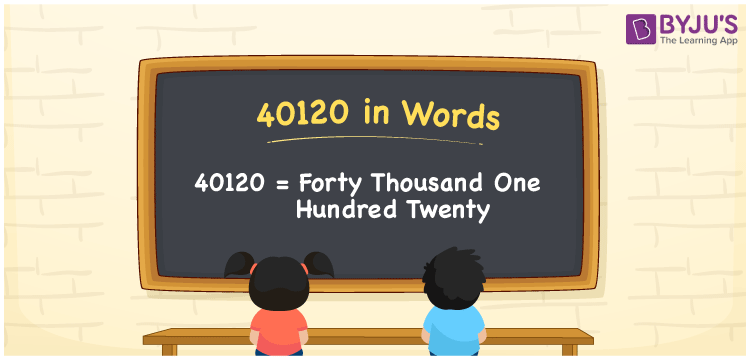# 40120 in Words

40120 in words is written as Forty thousand one hundred twenty. In both the International System of Numerals and the Indian System of Numerals, 40120 is written as Forty thousand one hundred twenty. The number 40120 is a Cardinal Number as it could represent some quantity. For example, “that laptop costs 40120 rupees”.

 40120 in Words Forty thousand one hundred twenty Forty thousand one hundred twenty in Number 40120

## 40120 in English Words

40120 in English words is read as “Forty thousand one hundred twenty”.## How to Write 40120 in Words?

To write 40120 in words, we shall use the place value chart. In the place value chart, put 4 in the ten thousands, 0 in the thousands, 1 in the hundreds, 2 in the tens and 0 in the ones. Let us make a place value chart to write the number 40120 in words.

 Ten Thousands Thousands Hundreds Tens Ones 4 0 1 2 0

Thus, we can write the expanded form as

4 × Ten Thousand + 0 × Thousand + 1 × Hundred + 2 × Ten + 0 × One

= 4 × 10000 + 0 × 1000 + 1 × 100 + 2 × 10 + 0 × 1

= 40000 + 0 + 100 + 20 + 0

= 40120

= Forty thousand one hundred twenty.

40120 is a natural number, the successor of 40119 and the predecessor of 40121.

40120 in words – Forty thousand one hundred twenty

• Is 40120 an odd number? – No
• Is 40120 an even number? – Yes
• Is 40120 a perfect square number? – No
• Is 40120 a perfect cube number? – No
• Is 40120 a prime number? – No
• Is 40120 a composite number? – Yes

## Frequently Asked Questions on 40120 in Words

Q1

### How to write 40120 in words?

40120 in words is written as Forty thousand one hundred twenty.
Q2

### How to write 40120 in the International and Indian System of Numerals?

In both, the system of numerals, 40120 in words, is written as Forty thousand one hundred twenty.
Q3

### How to write 40120 in a place value chart?

In the place value chart, write 4 in the ten thousands, 0 in the thousands, 1 in the hundreds, 2 in the tens and 0 in the ones.